Next: Polarization efficiency: size/shape/orientation effects Up: Optical properties of cosmic Previous: The  2175Å feature

To model the interstellar polarization one needs to calculate the forward-transmitted radiation for an ensemble of non-spherical aligned dust grains. This procedure consists of two steps: 1) computations of the extinction cross-sections for two polarization modes and 2) averaging of cross-sections for given particles size and orientation distributions. Although the average cross-sections should be compared with observations, behaviour of the polarization cross-sections and alignment mechanisms are often analysed separately.

Let non-polarized stellar radiation passes through a dusty cloud with a homogeneous magnetic field. As follows from observations and theoretical considerations (Dolginov et al., ), the magnetic field determines the direction of alignment of dust grains. The angle between the line of sight and the magnetic field is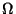(). The linear polarization produced by a rotating spheroidal particle of same size is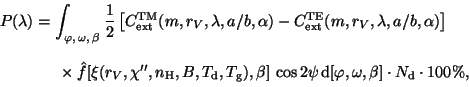whereare the extinction cross-sections for two polarization modes (Sect.),the column density of dust grains. The particles are assumed to be partially aligned: the major axis of the particle rotates in the spinning plane (is the spin angle) which is perpendicular to the angular momentum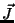.spins (precesses) around the direction ofmagnetic field (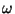is the precession angle),is the precession-cone angle for. This is the imperfect Davies-Greenstein (IDG) orientation described by the functionwhich depends on the alignment parameter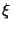and the angle.

Note that the problem of grain alignment is one of the most difficult in the physics of cosmic dust. Here, the interaction of solid particles with gas, radiation and magnetic field is closely connected. Davies and Greenstein () assumed that Fe atoms embedded in dielectric particles gave them paramagnetic properties and opened the possibility of interaction with a weak interstellar magnetic field. The required orientation arises as a result of the effect of paramagnetic relaxation of thermally rotating grains. The Davies-Greenstein mechanism was further developed by Jones and Spitzer () who obtained expressions for the distribution of angular momentum. In the simplest case, it is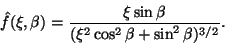(8)

The parameteris a function of the particle size, the imaginary part of the grain magnetic susceptibility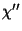(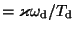, whereis the angular velocity of grain), gas density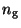, the strength of magnetic fieldand dust (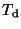) and gas (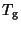) temperatures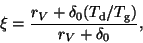(9)

where(10)

The anglein Eq. (8) is expressed via the anglesand(for definitions of the angles and relations between them see, for example, Hong and Greenberg,  or Voshchinnikov, ).

Rotation is an important factor of any grain alignment mechanism. The faster it is the more effective the grain alignment should be. The Davies-Greenstein mechanism considers thermally rotating grains. Purcell () suggested a mechanism of supra-thermal spin alignment (SSA; pinwheel'' mechanism) where the grains were spun up to very high velocities as a result of the desorption of Hmolecules from their surfaces. In this case, the alignment function is described by Eq. (9) but the parameter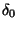is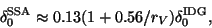(11)

whereis in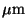. Rotation can also arise due to radiation torques when helical'' grains scatter left- and right-circularly polarized light in a different way (Dolginov et al., ; Draine and Weingartner, , ), which can lead to the grain alignment in anisotropic radiation field. Alignment of thermally rotating grains is also possible by supersonic flows or Alfvénic waves or ambipolar diffusion. This so-called Gold-type3 of mechanical alignment was generalized for supra-thermally rotating grains by Lazarian (). Note that some mechanisms can produce alignment when the major grain axes tend to align parallel to the magnetic field. Such an orientation is wrong'' for interstellar polarization but the mechanism may be right'' and operate in other conditions, for example in jets from YSO.

The development and current status of the major alignment mechanisms and principal physical processes forming their basis are reviewed by Roberge (), Lazarian et al. () and Lazarian (). Unfortunately, the astrophysical significance of different alignment mechanisms remains unclear. This is connected, in particular, with very rough theoretical estimates of the polarization efficiency when instead of an alignment function like that given by Eq. (9) the Rayleigh reduction factor (see Greenberg, ) is used (e.g., Lazarian et al., ).

The circular polarization is proportional to the product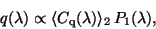(12)

whereis the average cross-section of circular polarization. The cross-section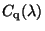is calculated as the difference of phase lags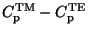represented by the imaginary parts of the corresponding complex extinction cross-sections. The circular polarization arises when the radiation linearly polarized in cloud 1'' passes through cloud 2'' (note the indices in Eq. (13)). Cloud 1'' works as a linear polarizer and cloud 2'' as a linear retarder. In such an optical device, the maximum transformation of the linear polarization to the circular one occurs when the optical axes of components are inclined by 45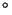(e.g., Tinbergen, ).

For simplicity, non-rotating particles of the same orientation are frequently considered. In this case of picket fence'' (PF) orientation, there are no integrals over angles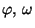andin Eq. (8). The polarization degree is proportional to the polarization cross-section, where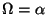. The dichroic polarization efficiency is defined by the ratio of the polarization cross-section (factor) to the extinction one(13)

where the upper (lower) sign is related to prolate (oblate) spheroids. This ratio describes the efficiency of polarization of light transmitted through an uniform slab consisting of non-rotating particles of the same orientation.

A more complicated case is the perfect rotational (2D) orientation (or perfect Davies-Greenstein orientation, PDG) when the major axis of a non-spherical particle always lies in the same plane. For the 2D orientation, integration is performed over the spin angleonly. This gives for prolate spheroids(14)

where the angle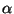is connected withand(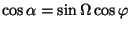). For oblate spheroids randomly aligned in a plane, we haveand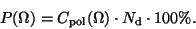(15)

As a result, the expected polarization will be determined by:

• the particle refractive index, size and shape via the polarization cross-section;
• the relation between the strength of the magnetic field, gas and dust temperature, gas density, etc. via the alignment function;
• the direction of alignment depending on the angle(or) via bothand.
The simplest types of orientations like PF or PDG allow one to investigate the influence of the first and third factors. The dependence of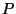onis excluded because the normalized polarization or polarization efficiency is usually studied.

SubsectionsNext: Polarization efficiency: size/shape/orientation effects Up: Optical properties of cosmic Previous: The  2175Å feature
root 2003-04-09